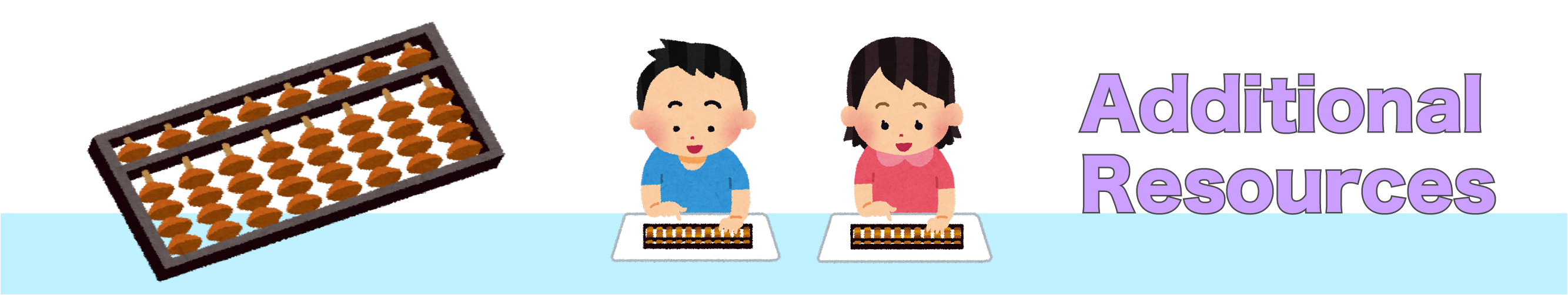Subject: Maths
Level: Key Stage 1 / Key Stage 2
Age: 5-7 years / 7-11 years
Resource Type: WorksheetsThe resources below are supplementary to the scheme of work. There are worksheets which can be used to provide students with more practice reading numbers on the soroban, and worksheets with three digit numbers for older or more confident students.

This printout template can be used for students to practice soroban at home.

Students order the numbers shown on the soroban from smallest to largest (up to 9). This worksheet can be used after Lesson 1.

Students order the numbers shown on the soroban from smallest to largest (up to 99). This worksheet can be used after Lesson 2.

Students read the numbers on the soroban and write in the number that is next in the sequence. Note: This is for reading practice and students will not be able to work out the sequence using the soroban yet.

#### Worksheets with 3-digit numbers:

Students order the numbers shown on the soroban from smallest to largest (up to 999).

Students read the soroban (3-digit numbers) and draw a greater or less than symbol in the middle box.

Students add the numbers shown on the soroban (3 digit-numbers)

<< Back to Main Soroban Resources Page